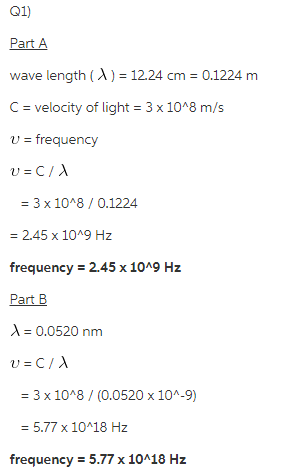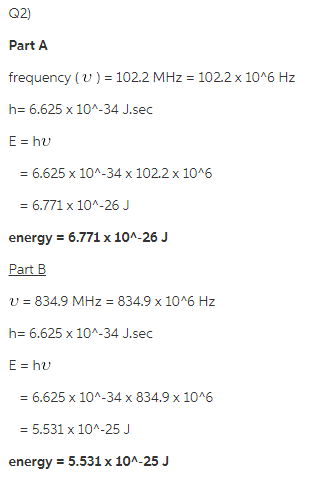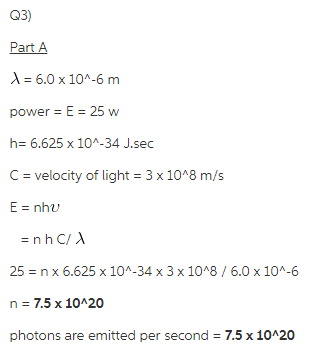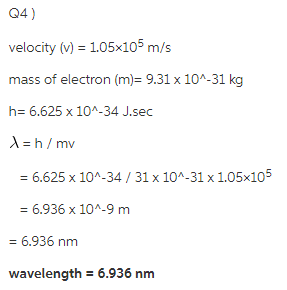# Calculate the frequency of each of the following wave lengths of electromagnetic radiation?

Q1)Calculate the frequency of each of the following wave lengths of electromagnetic radiation.

Part A
12.24 cm (wavelength of microwave oven)

Part B
0.0520 nm (a wavelength contained in medical X-rays)

Q2)Calculate the energy of a photon of electromagnetic radiation at each of the following frequencies.
Part A

Part B
834.9 MHz (common frequency used for cell phone communication)

Q3)A heat lamp produces 25.0 watts of power at a wavelength of 6.0 μm .

Part A
How many photons are emitted per second? (1 watt = 1 J/s)

Q4)What is the de Broglie wavelength of an electron traveling at 1.05×105 m/s ?